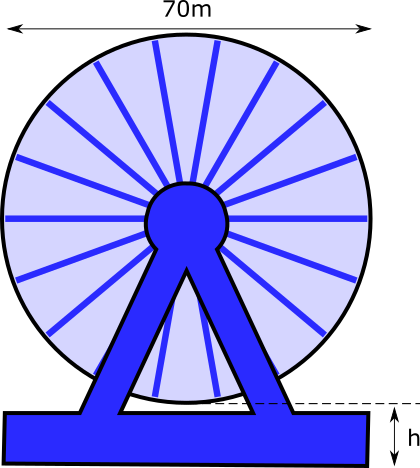# Exam-Style Questions on Modelling

## Problems on Modelling adapted from questions set in previous Mathematics exams.

### 1.

IB Applications and Interpretation

In a fantasy story the power value of a dream catcher varies depending on its length. The power values of various dream catchers are recorded in the following table:

 Length, $$x$$ cm 0 10 15 Power, $$p$$ W 0 12 22

This information was used to create Model A, where $$p$$ is a function of $$x$$ , $$x \ge 0$$.

Model A: $$p(x) = ax^2 + bx$$ , where $$a,b \in \mathbb{Z}$$.

When the length is 10 cm, Model A can be represented by the equation 50a + 5b = 6.

(a) Write down a second equation to represent Model A, when the length is 15cm.

(b) Find the values of a and b.

(c) Find the coordinates of the vertex of the graph of $$y = p(x)$$.

(d) Using the values in the table and your answer to part (c), sketch the graph of $$y = p(x)$$ for $$0 \le x \le 15$$ and $$0 \le p \le 22$$.

Additional data was used to create Model B, a revised model for the power of a dream catcher.

Model B: $$p(x) = 0.06x^2 + 0.68x$$

(e) Use Model B to calculate an estimate for the power of a dream catcher of length 18cm.

The actual power of a dream catcher of length 18cm is 30 W.

(f) Calculate the percentage error in the estimate in part (e).

### 2.

IB Standard

A Big Wheel at an amusement park has a diameter of length 70 metres which rotates at a constant speed. The bottom of the wheel is h metres above the ground. A seat starts at the bottom of the wheel.The wheel completes one revolution in 4 minutes. [The diagram is not to scale](a) After 2 minutes, the seat is 76m above the ground. Find h.

(b) After t minutes, the height of the seat above ground is given by $$f(t) =41+ k \cos{ \frac{\pi t}{2}}$$ for $$0 \le t \le 16$$.
Find the value of k.

(c) Find when the seat is 40 m above the ground for the third time.

If you would like space on the right of the question to write out the solution try this Thinning Feature. It will collapse the text into the left half of your screen but large diagrams will remain unchanged.

The exam-style questions appearing on this site are based on those set in previous examinations (or sample assessment papers for future examinations) by the major examination boards. The wording, diagrams and figures used in these questions have been changed from the originals so that students can have fresh, relevant problem solving practice even if they have previously worked through the related exam paper.

The solutions to the questions on this website are only available to those who have a Transum Subscription.

Exam-Style Questions Main Page

Search for exam-style questions containing a particular word or phrase:

To search the entire Transum website use the search box in the grey area below.